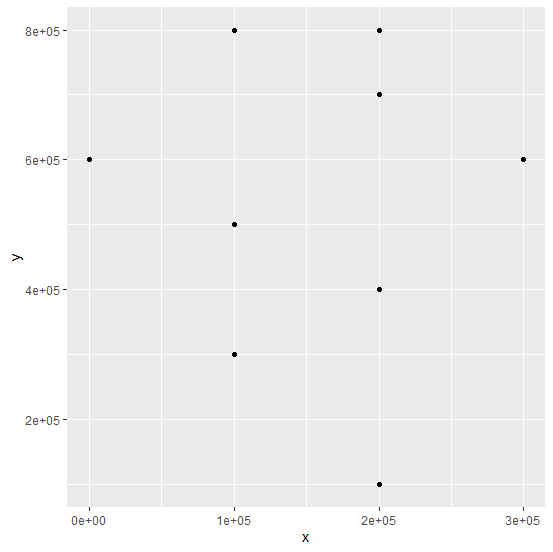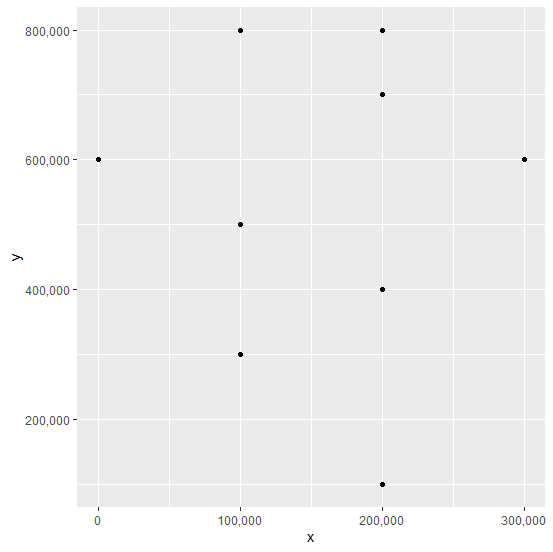# How to change number formatting from scientific to numbers of axes labels in a scatterplot using ggplot2 package in R?

R ProgrammingServer Side ProgrammingProgramming

When we create a scatterplot or any other plot and the values are presented in scientific form in the original data then the axes values of the plot are also plotted in scientific form. This makes the plot ambiguous, therefore, reading the plot or interpreting it becomes difficult. Hence, we need to convert the scientific form of the axes labels to numbers and it can be done by using scale_x_continuous(labels =comma) and scale_y_continuous(labels=comma) for both the axes.

## Example

Consider the below data frame −

set.seed(101)
x <-rpois(10,2)*100000
y <-rpois(10,5)*100000
df <-data.frame(x,y)
library(ggplot2)

Creating the scatterplot −

ggplot(df,aes(x,y))+geom_point()

## OutputCreating the scatterplot with numbers on axes −

## Example

ggplot(df,aes(x,y))+geom_point()+scale_x_continuous(labels = comma)+scale_y_continuous(labels=comma)

## Output